# Ecological Sampling Measuring biotic components of a system

• Slides: 17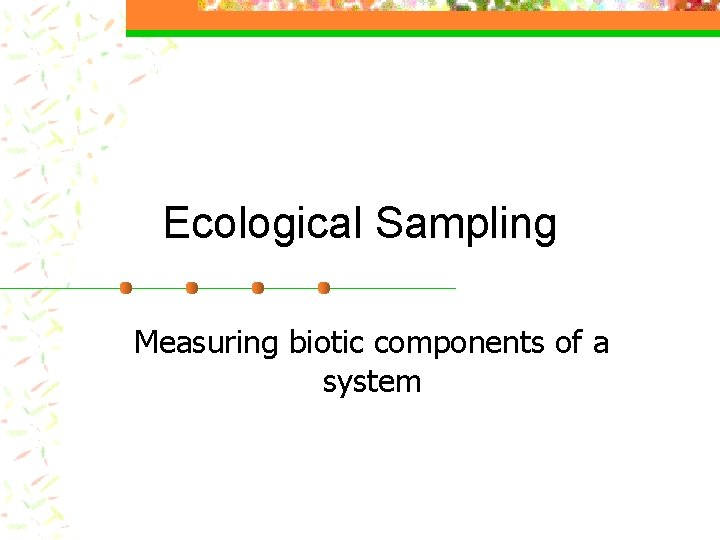Ecological Sampling Measuring biotic components of a systemEcological Sampling n What is a sample? n “A portion, piece, or segment that is representative of a whole” n Why do we sample? n it is usually impossible to measure the wholeOne big assumption… n n n That the sample is representative of the whole It is necessary to take enough samples so that an accurate representation is obtained It is important to avoid bias when sampling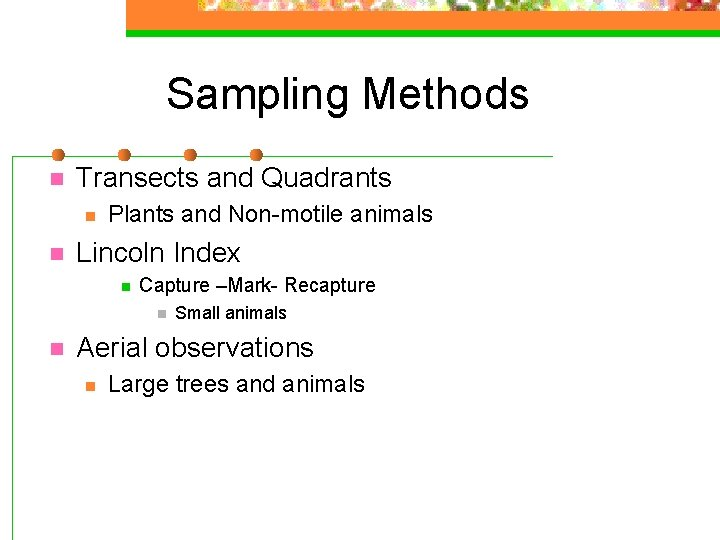Sampling Methods n Transects and Quadrants n n Plants and Non-motile animals Lincoln Index n Capture –Mark- Recapture n n Small animals Aerial observations n Large trees and animalsSampling along Transects n n Samples taken at fixed intervals Set up along an environmental gradient (e. g. high to low on a mountain)Line transect method n n n A measured line laid across the area in the direction of the environmental gradient All species touching the line are be recorded along the whole length of the line or at specific points along the line Measures presence or absence of species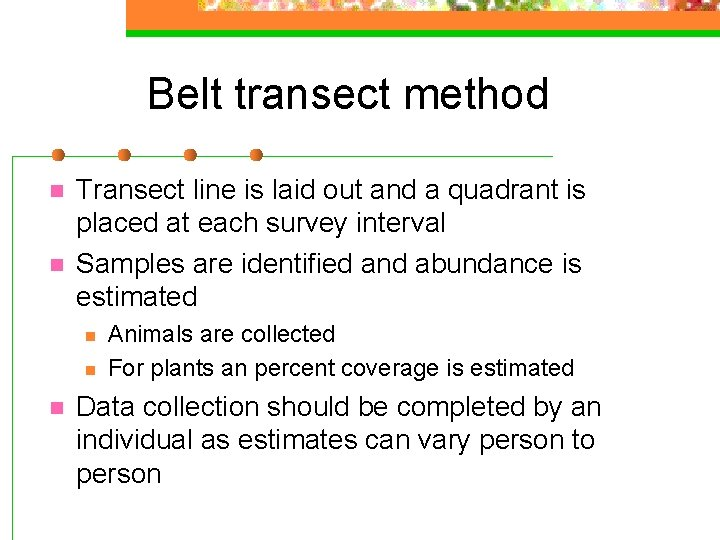Belt transect method n n Transect line is laid out and a quadrant is placed at each survey interval Samples are identified and abundance is estimated n n n Animals are collected For plants an percent coverage is estimated Data collection should be completed by an individual as estimates can vary person to person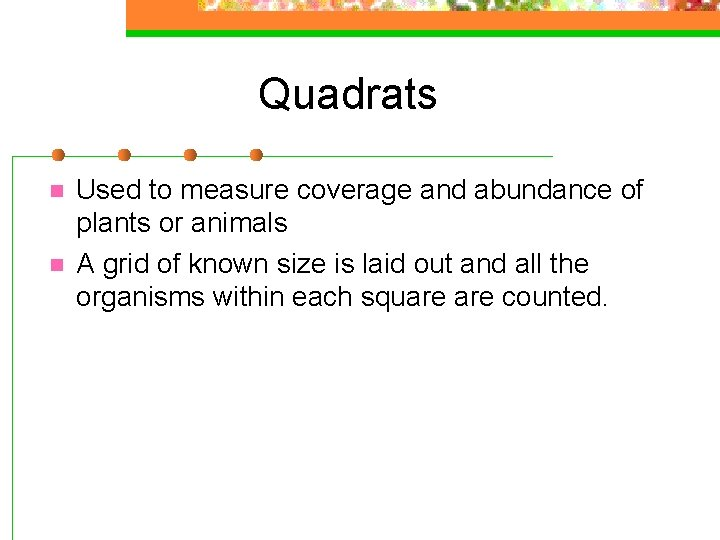Quadrats n n Used to measure coverage and abundance of plants or animals A grid of known size is laid out and all the organisms within each square counted.Lincoln Index n Capture-Mark-Recapture n n Animals are captured, counted, tagged and released. After a period of time another capture occurs. Previously tagged animals are counted and unmarked organisms are marked. Abundance is calculated using the following formula: n 1 x n 2 n =total marked after catch 1 n 3 1 n 2=total marked after catch 2 n 3=total caught in catch 2 but marked in catch 1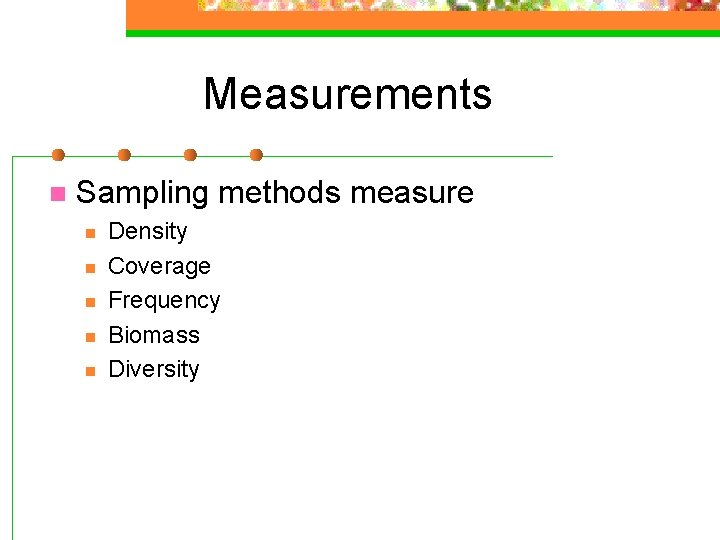Measurements n Sampling methods measure n n n Density Coverage Frequency Biomass DiversityDensity (D) n The number of individuals per unit area n n =number of individuals for i D=ni/A species i Eg. 10 dandelions/m 2 A=the area sampled (could Relative density i (Rdi)be the volume V) n n n The Density of species i, Divided by the sum of all the densities of the other species sampled Rdi=Di/S D Eg. 10/5+8+16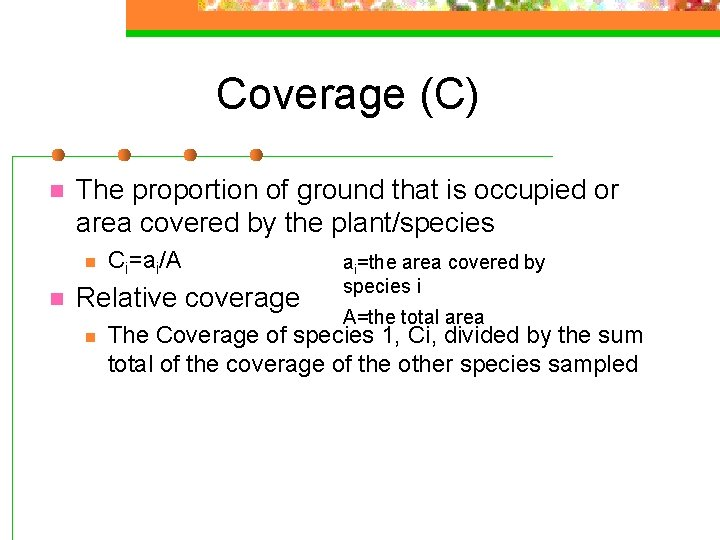Coverage (C) n The proportion of ground that is occupied or area covered by the plant/species n n Ci=ai/A Relative coverage n ai=the area covered by species i A=the total area The Coverage of species 1, Ci, divided by the sum total of the coverage of the other species sampled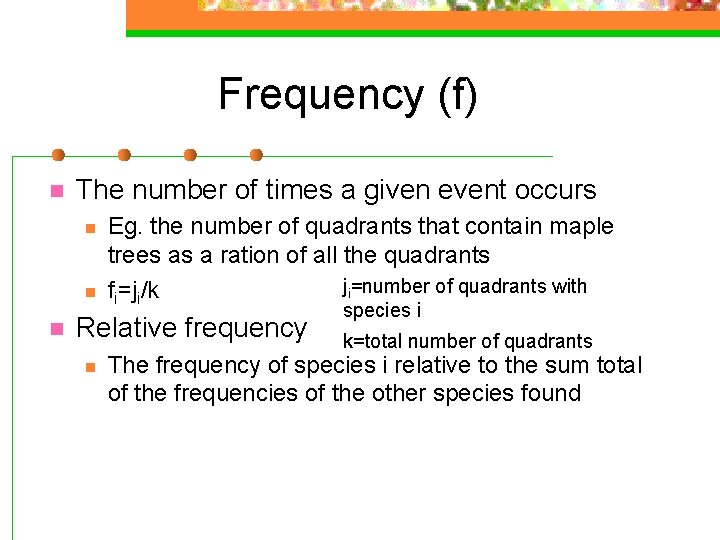Frequency (f) n The number of times a given event occurs n n n Eg. the number of quadrants that contain maple trees as a ration of all the quadrants ji=number of quadrants with fi=ji/k Relative frequency n species i k=total number of quadrants The frequency of species i relative to the sum total of the frequencies of the other species found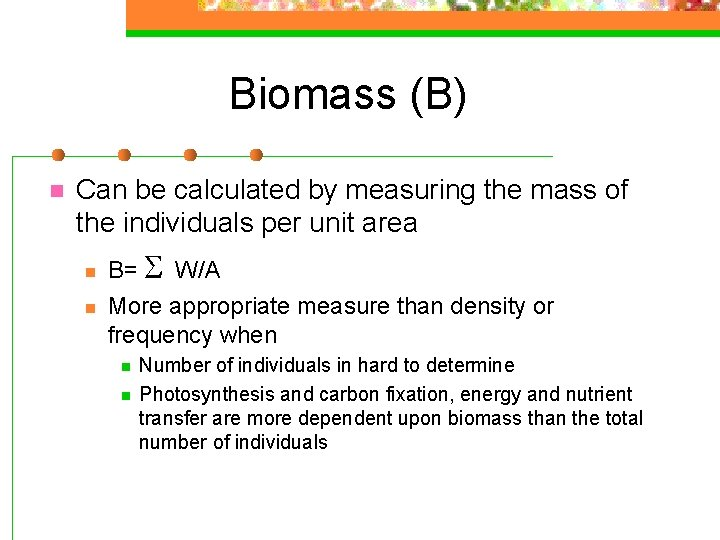Biomass (B) n Can be calculated by measuring the mass of the individuals per unit area n n B= S W/A More appropriate measure than density or frequency when n n Number of individuals in hard to determine Photosynthesis and carbon fixation, energy and nutrient transfer are more dependent upon biomass than the total number of individuals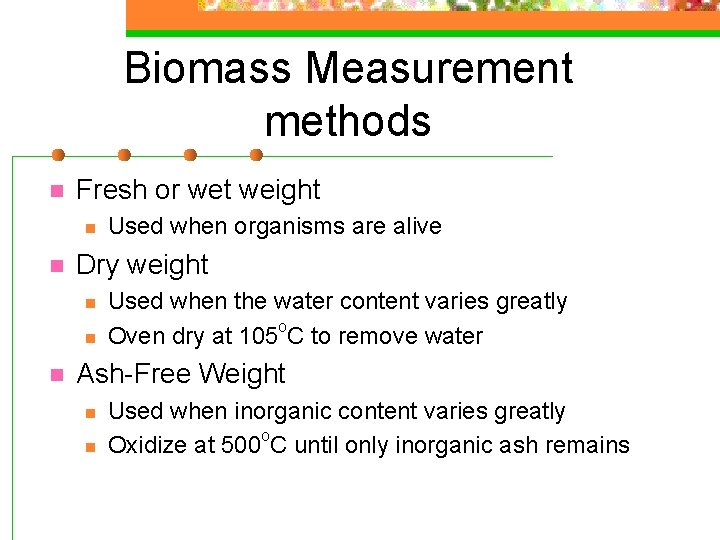Biomass Measurement methods n Fresh or wet weight n n Dry weight n n n Used when organisms are alive Used when the water content varies greatly Oven dry at 105 o. C to remove water Ash-Free Weight n n Used when inorganic content varies greatly Oxidize at 500 o. C until only inorganic ash remains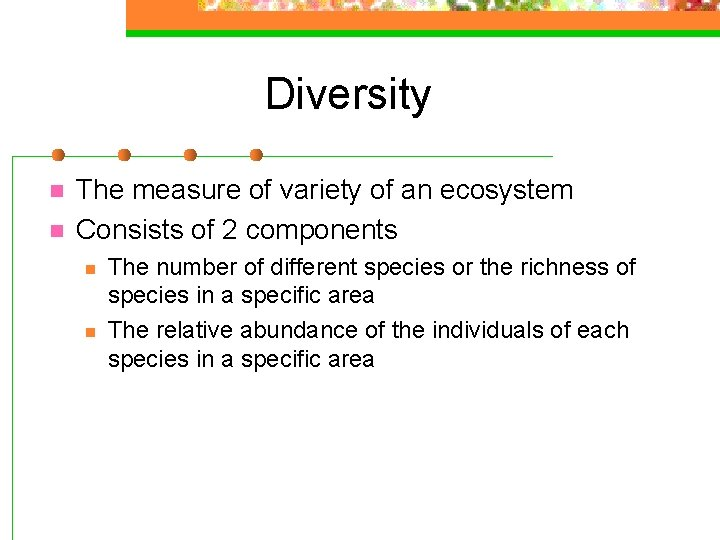Diversity n n The measure of variety of an ecosystem Consists of 2 components n n The number of different species or the richness of species in a specific area The relative abundance of the individuals of each species in a specific areaSimpson's Diversity (D) n Measures species richness D=N(N-1) S n(n-1) D=Diversity N=total number of organisms of all species found n=number of individuals of a particular species n n If D is high the area may be a stable ancient site. Low D may suggest pollution, recent colonization, or agricultural management